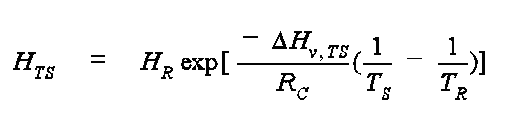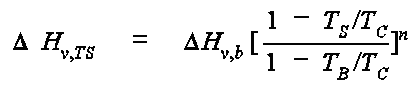# EPA On-line Tools for Site Assessment Calculation

Two methods are used to calculate the temperature-dependence of Henry's law coefficients. First is a method used to adjust the values using the Clausius-Clapeyron relationship:where HTS is the dimensional (atm-m3/mol) Henry's Law coefficient at the Kelvin temperature, TS, DHv, TS is the enthalpy of vaporization at TS in units of cal/mol, TR is the reference temperature for Henry's Law (HR) in K, and RC is the gas constant and is equal to 1.9872 cal/mol-K. The enthalpy of vaporization, DHv, TS is estimated fromwhere DHv, b is the enthalpy of vaporization at the normal boiling point (cal/mol), TB is the normal boiling point in K, and TC is the critical temperature in K. The exponent n is selected from the following table:

 Ratio TB/TC Exponent n < 0.57 0.30 0.57 to 0.71 0.74 (TB/TC) - 0.116 > 0.71 0.41

All of the input values required for these equations were generated by OSWER and are available in a fact sheet (PDF, 9 pp., 0.186 MB, About PDF). The fact sheet contains more information on these methods. Notably the normal boiling point enthalpies of vaporization (DHv, b) were estimated from the Antoine equation for vapor pressure. The coefficients of the Antoine equation where themselves estimated from methods given in the factsheet. These quantities are not recalculated in the Henry's law calculator because they were tabulated in the attachment to the factsheet.

The second method was developed by Washington (1996)1 for many compounds reported in this calculator, variation of Henry's Law Constant with temperature is quantified by the van't Hoff Equation:where ln Kh is the natural logarithm of the Henry's constant
D Hr qis the standard state enthalpy
R is the universal gas constant
T is the temperature in Kelvin, and
DSr qis the standard state entropy.

This method is based upon the assumption that the head capacity is the same for the reactants and products. Therefore the temperature range is not extrapolated beyond values used in generating the coefficients used in making the estimates.

1 Washington, J., 1996, Gas partitioning of dissolved volatile organic compounds in the vadose zone: Principles, temperature effects and literature review, Ground Water, 34(4), 709-718.

 Top ^ Home | Glossary | Notation | Links | References | Calculators

Contact Jim Weaver to ask a technical question on this material.